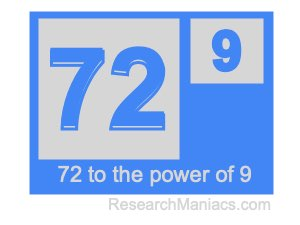What is 72 to the power of 9?Here we will show you the answer to 72 to the power of 9 (seventy-two to the power of nine) and explain how we got the answer.

To find the answer, you multiply 72 by itself 9 times as follows:

72 × 72 × 72 × 72 × 72 × 72 × 72 × 72 × 72 =

And when we solve the problem above, we get the answer as follows:

51,998,697,814,228,992

Note that 72 to the power of 9 is the same as 72 raised to 9. In xy, 72 is the base (x) and 9 is the exponent (y). Therefore, you can also write the problem and the answer as follows:

729=51,998,697,814,228,992

You will also get the answer of 72 to the power of 9 (72 to the 9th power) if you type 72 then xy then 9 and then = on your scientific calculator.

Power of Calculator
Enter another base (x) and exponent (y) below to solve another xy problem.

to the power of

What is 72 to the power of 10?
Go here for the next "power of" calculation we have done for you.